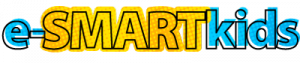# What’s a Watt? Activity Tips

The What’s a Watt? activity helps students explore the concepts of amps, volts, and watts and how they relate to some common, everyday appliances.

Getting It Across

Discuss with students the definitions of amps, volts, watts, and megawatts.

Amps: The measure of the amount of electricity used.

Volts: The measure of the pressure or force of electricity.

Watts: Amps multiplied by the volts gives you the watts. This is the measure of the work that electricity does per second.

Megawatts: One million watts.

This activity asks students to use the formula amps x volts = watts to figure out how many watts these typical appliances use. Most homes are served by 120-volt electricity, so students are asked to use that as the value for volts in their equations.

Extension

• Have students research what types of appliances in their homes might use very few watts (clock, flashlight, or phone) or lots of watts (clothes dryer, oven, or central air conditioner) and report back to the class.
• Discuss with students when megawatts might be used. (For measuring the watts used by cities or generated by power plants.)